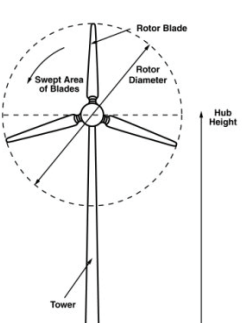•### - Measuring the Swept Area of Your Wind Turbine

Being able to measure the swept area of your blades is essential if you want to analyze the efficiency of your wind turbine.
The swept area refers to the area of the circle created by the blades as they sweep through the air.
To find the swept area, use the same equation you would use to find the area of a circle can be found by following
equation:
Area =πr2
-
π = 3.14159 (pi)
r = radius of the circle. This is equal to the length of one of your blades.
-
-
-
-### - Why is This Important ?

You will need to know the swept area of your wind turbine to calculate the total power in the wind that hits your turbine.
Remember the Power In The Wind Equation:
P = 1/2 x ρ x A x V3
-
P = Power (Watts)
ρ = Air Density (about 1.225 kg/m3 at sea level)
A = Swept Area of Blades (m2 )
V = Velocity of the wind
-
-
By doing this calculation,  you can see the total energy potential in a given area of wind. You can then compare this to the actual amount of power you are producing with your wind turbine (you will need to calculate this using a multimeter—multiply voltage by amperage).
The comparison of these two figures will indicate how efficient your wind turbine is.
Of course, finding the swept area of your wind turbine is an essential part of this equation!

Post time: Apr-18-2023